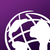# ArcPy Error : Can't Multiply Sequence by non-int of type 'list

1940
6
02-21-2017 12:30 PMOccasional Contributor III

Hi,

I am trying to execute an equation into python program considering monthly folder, but I am getting error, Below i have attached reference of the equation also, Where P and R  for rasterlist1 and rasterlist2 respectively,

Error message is.

``````Traceback (most recent call last):
File "<module3>", line 23, in <module>
TypeError: can't multiply sequence by non-int of type 'list'‍‍‍‍‍‍‍‍‍‍‍‍``````

Script i am working,

``````import arcpy, os, calendar
import numpy
from arcpy.sa import *
arcpy.env.overwriteOutput = True
arcpy.CheckOutExtension("Spatial")
topWorkspace = r'D:\NPP_GUJARAT_MONSOON'
ws_out = r'D:\NPP_GUJARAT_MONSOON\EVA3'
arcpy.env.workspace = topWorkspace

months = {calendar.month_name[i].upper(): str(i).zfill(3) for i in range(1, 13)} # Get dict of months and month number (i.e. January = 001, March = 003 etc.)

# Step through list of all folders
for folderPath in arcpy.ListWorkspaces():
baseName = os.path.basename(folderPath).upper()
if baseName in months: # Test that subfolder is a month name
monthNumber = months[baseName] # Get month-number for use in output filename
arcpy.env.workspace = folderPath

# list rasters (Erlier:*Max_Temper.tif, We changed it to below)
rasterList1 = arcpy.ListRasters('Precipitat*.tif')
rasterList2 = arcpy.ListRasters('Solar*.tif')
print rasterList1, rasterList2

for ras_name in rasterList1:
ras_mean = arcpy.Raster(os.path.join(folderPath, ras_name))

for ras_name in rasterList2:
ras_mean = arcpy.Raster(os.path.join(folderPath, ras_name))

outCellStatistics = ((rasterList1 * rasterList2) * ((Square(rasterList1)) + (Square(rasterList2)) + rasterList1 * rasterList2)) /rasterList1 + rasterList2 * ((Square(rasterList1)) + (Square(rasterList2)))

#Save the output
outRasterName = os.path.join(ws_out, "EO_{}.tif".format(monthNumber))
outCellStatistics.save(outRasterName)
print outRasterName

#print done
print 'done'
‍‍‍‍‍‍‍‍‍‍‍‍‍‍‍‍‍‍‍‍‍‍‍‍‍‍‍‍‍‍‍‍‍‍‍‍‍‍‍‍‍‍``````Tags (4)
6 RepliesbyMVP Honored Contributor

You can't multiply lists of rasters together - it just doesn't work:

``````>>> arcpy.env.workspace = r'C:\junk'
... rasterlist = arcpy.ListRasters()
... newlist = rasterlist * rasterlist
...
Runtime error
Traceback (most recent call last):
File "<string>", line 3, in <module>
TypeError: can't multiply sequence by non-int of type 'list'‍‍‍‍‍‍‍‍``````byMVP Legendary Contributor

Times in SA there are other cell-by-cell functions there as wellbyMVP Legendary Contributor

You may have been thinking of numpy as in some previous threads...

``````a = np.arange(9).reshape(3,3)

b = a[::-1]

a
Out:
array([[0, 1, 2],
[3, 4, 5],
[6, 7, 8]])

b
Out:
array([[6, 7, 8],
[3, 4, 5],
[0, 1, 2]])

a*b
Out:
array([[ 0,  7, 16],
[ 9, 16, 25],
[ 0,  7, 16]])‍‍‍‍‍‍‍‍‍‍‍‍‍‍‍‍‍‍‍‍‍‍‍‍‍‍‍‍‍‍‍‍‍‍‍‍‍‍‍‍‍‍``````

etcetera

RasterToNumPyArray and NumPyArrayToRaster should you need more that simple cell-by-cell arithmetic and other functionsOccasional Contributor III

Thank you for the suggestions. Again I have updated my full code. I think multiple sequence might be possible here. Please request to all cooperate with us to solve this issue.byMVP Legendary Contributor

Where did you update the code? I thought you just need to figure out how to multiply (aka, times) two rasters?

Did you not see the Plus and Pow(er) functions?

Provide your attempt at correcting it and any error messages you get.

Questions need to have some feedback to the answers, specifically what you tried and what worked and what didn't.Occasional Contributor III

Dan Patterson‌, Thank you very much. Finally, I reached to the end using below Script,

``````for ras_num in [str(i).zfill(3) for i in range(1, 13)]:

ras_Prcep = getRasterFromList(ras_num, lst_ras_Prcep, ws_in_Prcep)
ras_RD = getRasterFromList(ras_num, lst_ras_RD, ws_in_RD)

if all([not ras_Prcep is None, not ras_RD is None]):

# calculate
ras_E1A = (ras_Prcep * ras_RD) * ((Square(ras_Prcep)) + (Square(ras_RD) + ras_Prcep * ras_RD))

ras_E1B = ras_Prcep + ras_RD * ((Square(ras_Prcep)) + (Square(ras_RD)))

ras_AET = ras_E1A / ras_E1B
# save raster
out_name_AET = os.path.join(ws_out_AET, 'r{0}_AET.TIF'.format(ras_num))
ras_AET.save(out_name_AET)
print out_name_AET
‍‍‍‍‍‍‍‍‍‍‍‍‍‍‍‍‍‍‍‍‍‍‍‍‍‍‍‍‍‍‍‍‍‍‍‍‍‍‍‍‍‍‍‍‍‍‍‍‍‍‍‍‍‍‍‍‍‍‍‍‍‍‍‍‍‍‍‍‍‍‍‍‍‍‍‍‍‍‍‍‍‍‍‍‍‍‍‍‍‍‍‍‍‍‍‍‍‍‍‍‍‍‍‍``````